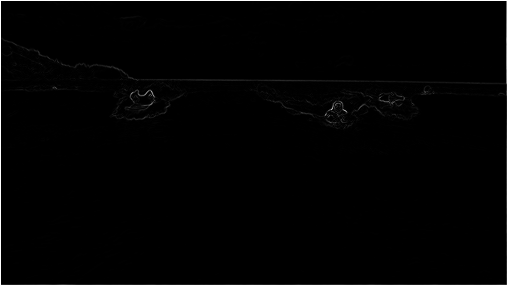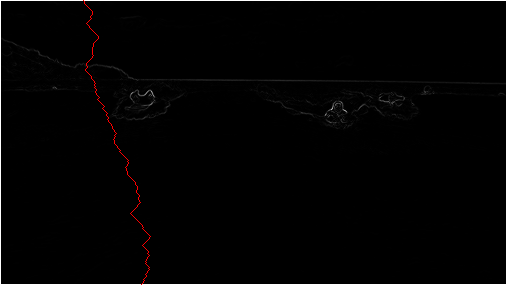## Programming Assignment 7: Seam Carving

Seam-carving is a content-aware image resizing technique where the image is reduced in size by one pixel of height (or width) at a time. A vertical seam in an image is a path of pixels connected from the top to the bottom with one pixel in each row; a horizontal seam is a path of pixels connected from the left to the right with one pixel in each column. Below left is the original 505-by-287 pixel image; below right is the result after removing 150 vertical seams, resulting in a 30% narrower image. Unlike standard content-agnostic resizing techniques (such as cropping and scaling), seam carving preserves the most interest features (aspect ratio, set of objects present, etc.) of the image.

The underlying algorithm is simple and elegant, but the technique was not discovered until 2007 by Shai Avidan and Ariel Shamir. Now, it is a core feature in Adobe Photoshop (thanks to a Princeton graduate student) and other computer graphics applications.In this assignment, you will create a data type that resizes a W-by-H image using the seam-carving technique. Finding and removing a seam involves three parts and a tiny bit of notation:

1. Notation. In image processing, pixel (x, y) refers to the pixel in column x and row y, with pixel (0, 0) at the upper left corner and pixel (W − 1, H − 1) at the bottom right corner. This is consistent with the Picture data type in stdlib.jar.

 (0, 0) (1, 0) (2, 0) (0, 1) (1, 1) (2, 1) (0, 2) (1, 2) (2, 2) (0, 3) (1, 3) (2, 3)

Warning: this is the opposite of the standard mathematical notation used in linear algebra where (i, j) refers to row i and column j and with Cartesian coordinates where (0, 0) is at the lower left corner.

We also assume that the color of each pixel is represented in RGB space, using three integers between 0 and 255. This is consistent with the java.awt.Color data type.

2. Energy calculation. The first step is to calculate the energy of a pixel, which is a measure of its importance—the higher the energy, the less likely that the pixel will be included as part of a seam (as we'll see in the next step). In this assignment, you will use the dual-gradient energy function, which is described below. Here is the dual gradient of the surfing image above:The energy is high (white) for pixels in the image where there is a rapid color gradient (such as the boundary between the sea and sky and the boundary between the surfing Josh Hug on the left and the ocean behind him). The seam-carving technique avoids removing such high-energy pixels.

3. Seam identification. The next step is to find a vertical seam of minimum total energy. This is similar to the classic shortest path problem in an edge-weighted digraph, but there are three important differences:

• The weights are on the vertices instead of the edges.

• The goal is to find the shortest path from any of the W pixels in the top row to any of the W pixels in the bottom row.

• The digraph is acyclic, where there is a downward edge from pixel (x, y) to pixels (x − 1, y + 1), (x, y + 1), and (x + 1, y + 1). assuming that the coordinates are in the prescribed ranges.

Seams cannot wrap around the image (e.g., a vertical seam cannot cross from the leftmost column of the image to the rightmost column).4. Finding a horizontal seam is analogous.

5. Seam removal. The final step is remove from the image all of the pixels along the vertical or horizontal seam.

The SeamCarver API. Your task is to implement the following mutable data type:

```public class SeamCarver {
public SeamCarver(Picture picture)                // create a seam carver object based on the given picture
public Picture picture()                          // current picture
public     int width()                            // width of current picture
public     int height()                           // height of current picture
public  double energy(int x, int y)               // energy of pixel at column x and row y
public   int[] findHorizontalSeam()               // sequence of indices for horizontal seam
public   int[] findVerticalSeam()                 // sequence of indices for vertical seam
public    void removeHorizontalSeam(int[] seam)   // remove horizontal seam from current picture
public    void removeVerticalSeam(int[] seam)     // remove vertical seam from current picture
}
```
• Corner cases. Your code should throw an exception when called with invalid arguments, as specified below.

• By convention, the indices x and y are integers between 0 and W − 1 and between 0 and H − 1 respectively, where W is the width of the current image and H is the height. Throw a java.lang.IndexOutOfBoundsException if either x or y is outside its prescribed range in energy().

• Throw a java.lang.NullPointerException if either removeVerticalSeam() or removeHorizontalSeam() is called with a null argument.

• Throw a java.lang.IllegalArgumentException if either removeVerticalSeam() or removeHorizontalSeam() is called with an array of the wrong length or if the array is not a valid seam (either an entry is outside the height/width bounds or two adjacent entries differ by more than 1).

• Throw a java.lang.IllegalArgumentException if either removeVerticalSeam() or removeHorizontalSeam() is called when the width or height of the current picture is 1, respectively.

• Constructor. The data type may not mutate the Picture argument to the constructor.
• Computing the energy of a pixel. You will use the dual-gradient energy function: The energy of pixel (x, y) is Δx2(x, y) + Δy2(x, y), where the square of the x-gradient Δx2(x, y) = Rx(x, y)2 + Gx(x, y)2 + Bx(x, y)2, and where the central differences Rx(x, y), Gx(x, y), and Bx(x, y) are the absolute value in differences of red, green, and blue components between pixel (x + 1, y) and pixel (x − 1, y). The square of the y-gradient Δy2(x, y) is defined in an analogous manner. To handle pixels on the borders of the image, calculate energy by defining the leftmost and rightmost columns as adjacent and the topmost and bottommost rows as adjacent. For example, to compute the energy of a pixel (0, y) in the leftmost column, we use its right neighbor (1, y) and its left neighbor (W − 1, y).

Consider the 3-by-4 image with RGB values (each component is an integer between 0 and 255) as shown in the table below.

 (255, 101, 51) (255, 101, 153) (255, 101, 255) (255,153,51) (255,153,153) (255,153,255) (255,203,51) (255,204,153) (255,205,255) (255,255,51) (255,255,153) (255,255,255)

• Non-border pixel example.   The energy of pixel (1, 2) is calculated from pixels (0, 2) and (2, 2) for the x-gradient

Rx(1, 2) = 255 − 255 = 0,
Gx(1, 2) = 205 − 203 = 2,
Bx(1, 2) = 255 − 51 = 204,
yielding Δx2(1, 2) = 22 + 2042 = 41620;

and pixels (1, 1) and (1, 3) for the y-gradient

Ry(1, 2) = 255 − 255 = 0,
Gy(1, 2) = 255 − 153 = 102,
By(1, 2) = 153 − 153 = 0,
yielding Δy2(1, 2) = 1022 = 10404.

Thus, the energy of pixel (1, 2) is 41620 + 10404 = 52024. Similarly, the energy of pixel (1, 1) is 2042 + 1032 = 52225.

• Border pixel example.   The energy of the border pixel (1, 0) is calculated by using pixels (0, 0) and (2, 0) for the x-gradient

Rx(1, 0) = 255 − 255 = 0,
Gx(1, 0) = 101 − 101 = 0,
Bx(1, 0) = 255 − 51 = 204,
yielding Δx2(1, 0) = 2042 = 41616;

and pixels (1, 3) and (1, 1) for the y-gradient

Ry(1, 0) = 255 − 255 = 0,
Gy(1, 0) = 255 − 153 = 102,
By(1, 0) = 153 − 153 = 0,
yielding Δy2(1, 2) = 1022 = 10404.

Thus, the energy of pixel (1, 2) is 41616 + 10404 = 52020.

 20808 52020 20808 20808 52225 21220 20809 52024 20809 20808 52225 21220

• Finding a vertical seam. The findVerticalSeam() method returns an array of length H such that entry i is the column number of the pixel to be removed from row i of the image. For example, consider the 6-by-5 image below (supplied as 6x5.png).

 ( 78,209, 79) ( 63,118,247) ( 92,175, 95) (243, 73,183) (210,109,104) (252,101,119) (224,191,182) (108, 89, 82) ( 80,196,230) (112,156,180) (176,178,120) (142,151,142) (117,189,149) (171,231,153) (149,164,168) (107,119, 71) (120,105,138) (163,174,196) (163,222,132) (187,117,183) ( 92,145, 69) (158,143, 79) (220, 75,222) (189, 73,214) (211,120,173) (188,218,244) (214,103, 68) (163,166,246) ( 79,125,246) (211,201, 98)

The corresponding pixel energies are shown below, with a minimum energy vertical seam highlighted in pink. In this case, the method findVerticalSeam() returns the array { 3, 4, 3, 2, 2 }.

 57685 50893 91370 25418 33055 37246 15421 56334 22808 54796 11641 25496 12344 19236 52030 17708 44735 20663 17074 23678 30279 80663 37831 45595 32337 30796 4909 73334 40613 36556

Remember that seams cannot wrap around the image. When there are multiple vertical seams with minimal total energy, your method can return any such seam.

• Finding a horizontal seam. The behavior of findHorizontalSeam() is analogous to that of findVerticalSeam() except that it returns an array of length W such that entry j is the row number of the pixel to be removed from column j of the image.

• Performance requirements. The width(), height(), and energy() methods should take constant time in the worst case. All other methods should run in time at most proportional to W H in the worst case.

Analysis of running time.  Estimate empirically the running times (in seconds) to remove one row and one column from a W-by-H image as a function of W, and H. Use tilde notation to simplify your answer. Answer the relevant questions in the readme.txt file.

Extra credit.

• Write a program SeamCarverGUI.java that resizes the image as the user resizes the window.

• Optimize SeamCarver.java so that you can run the algorithm at interactive speed for small images.

Submission.  Submit SeamCarver.java, and any other files needed by your program (excluding those in stdlib.jar and algs4.jar). Finally, submit a readme.txt file and answer the questions.

This assignment was developed by Josh Hug, Maia Ginsburg, and Kevin Wayne.